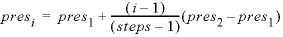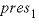| Next || Previous || Top |

## REACTION_PRESSURE

This keyword data block is used to define pressure during batch-reaction steps. This data block can also be used to specify the pressure in a cell or range of cells during advective-transport calculations ( ADVECTION ) and advective-dispersive transport calculations (TRANSPORT).

###### Example data block 1
`Line 0: REACTION_PRESSURE 1 Three explicit reaction pressures.`
`Line 1:     1.0     250.5     500.0`
###### Explanation 1

Line 0: REACTION_PRESSURE [ number ] [ description ]

REACTION_PRESSURE is the keyword for the data block.

number --Positive number or a range of numbers to designate this pressure definition. A range of numbers may be given in the form m-n , where m and n are positive integers, m is less than n , and the two numbers are separated by a hyphen without intervening spaces. Default is 1.

description --Optional comment that describes the pressure data.

Line 1: list of pressures

list of pressures --A list of pressures (atm) that will be applied to batch-reaction calculations. More lines may be used to supply additional pressures. One batch-reaction calculation will be performed for each listed pressure.

###### Example data block 2
`Line 0: REACTION_PRESSURE 1 Three implicit reaction pressures.`
`Line 1:      1.0     500.0 in 3 steps`
###### Explanation 2

Line 0: REACTION_PRESSURE [ number ] [ description ]

Same as Example data block 1.

Line 1: pres 1 , pres 2 , in steps

pres 1 --Pressure of first reaction step, atm.

pres 2 --Pressure of final reaction step, atm.

in steps --“ in ” indicates that the pressure will be calculated for each of steps number of steps. The pressure at each step, i , will be calculated by the formula; if steps = 1, then the pressure of the batch reaction will be. Example data block 2 performs exactly the same calculations as Example data block 1. If more batch-reaction steps are defined by KINETICS, REACTION, or REACTION_TEMPERATURE input, the pressure of the additional steps will be pres 2 .

###### Notes

If more batch-reaction steps are defined in KINETICS, REACTION, or REACTION_TEMPERATURE than pressure steps in REACTION_PRESSURE , then the final pressure will be used for all of the additional batch-reaction steps. INCREMENTAL_REACTIONS keyword has no effect on the REACTION_PRESSURE data block. The default pressure of a reaction step is equal to the pressure of the initial solution or the mixing-fraction-averaged pressure of a mixture. REACTION_PRESSURE input can be used even if there is no REACTION input. The method of calculation of pressure steps using “ in ” is slightly different than that for reaction steps. If n pressure steps are defined with “ in n ” in a REACTION_PRESSURE data block, then the pressure of the first reaction step is equal to pres 1 ; pressures in the remaining steps change in n-1 equal increments. In contrast, if n reaction steps are defined with “ in n ” in a REACTION data block, then the reaction is added in n equal increments.

In an advective-transport calculation ( ADVECTION ), if REACTION_PRESSURE n is defined (or a range is defined n - m ), and n is less than or equal to the number of cells in the simulation, then the first pressure in the data block of REACTION_PRESSURE n is used as the pressure in cell n (or cells n - m ) for all shifts in the advective-transport calculation. In advective-dispersive transport simulations (TRANSPORT), the initial equilibration also occurs at the first pressure of REACTION_PRESSURE n in cell n .

###### Example problems

The keyword REACTION_PRESSURE is used in example problem 2.

###### Related keywords

| Next || Previous || Top |